Chapter 5 Class 12 Continuity and Differentiability

Class 12
Important Questions for exams Class 12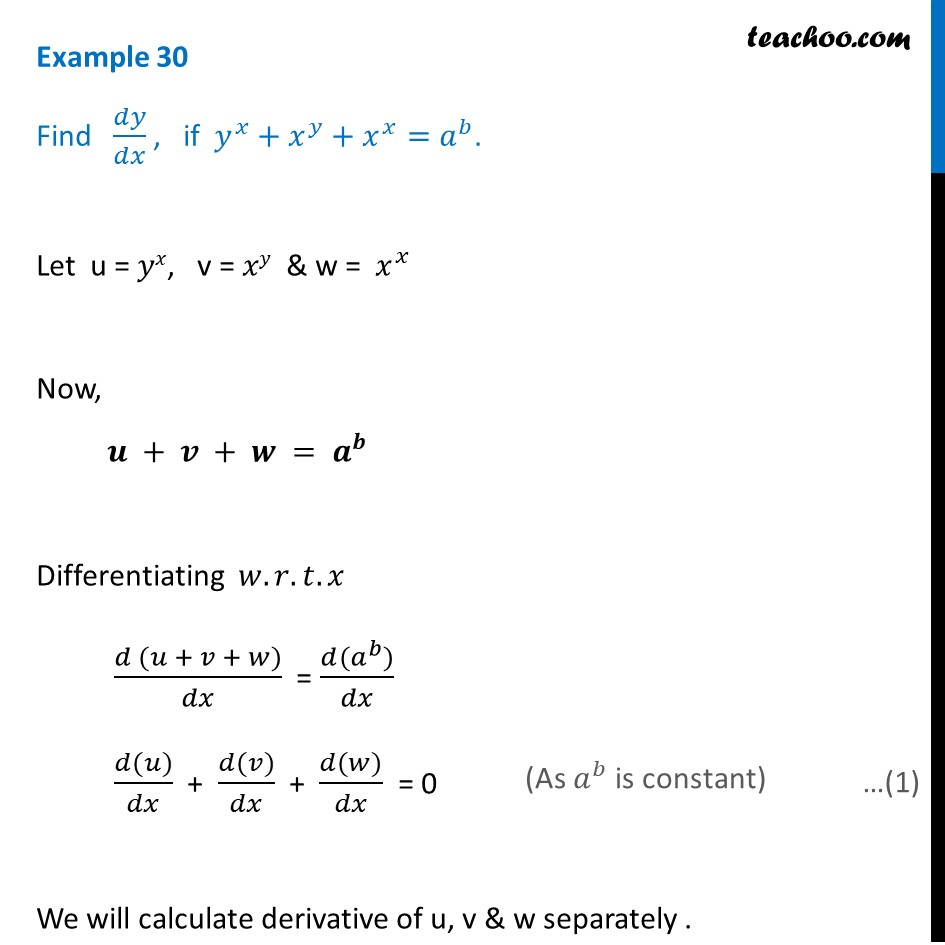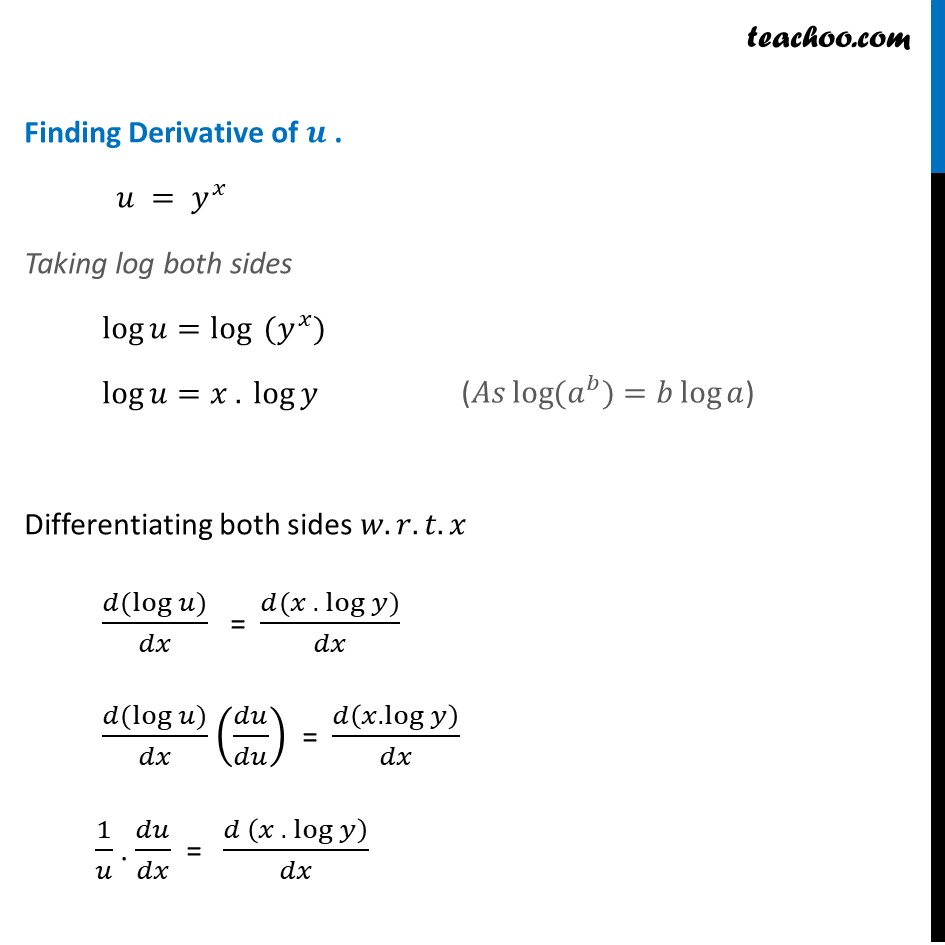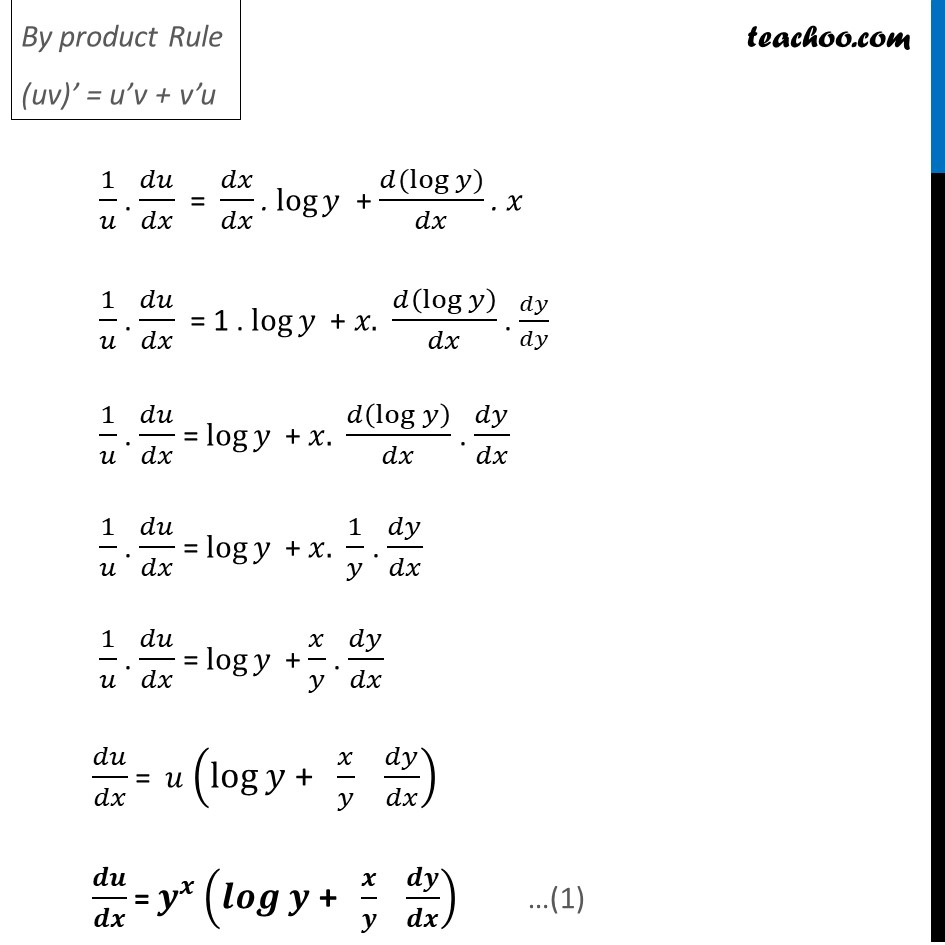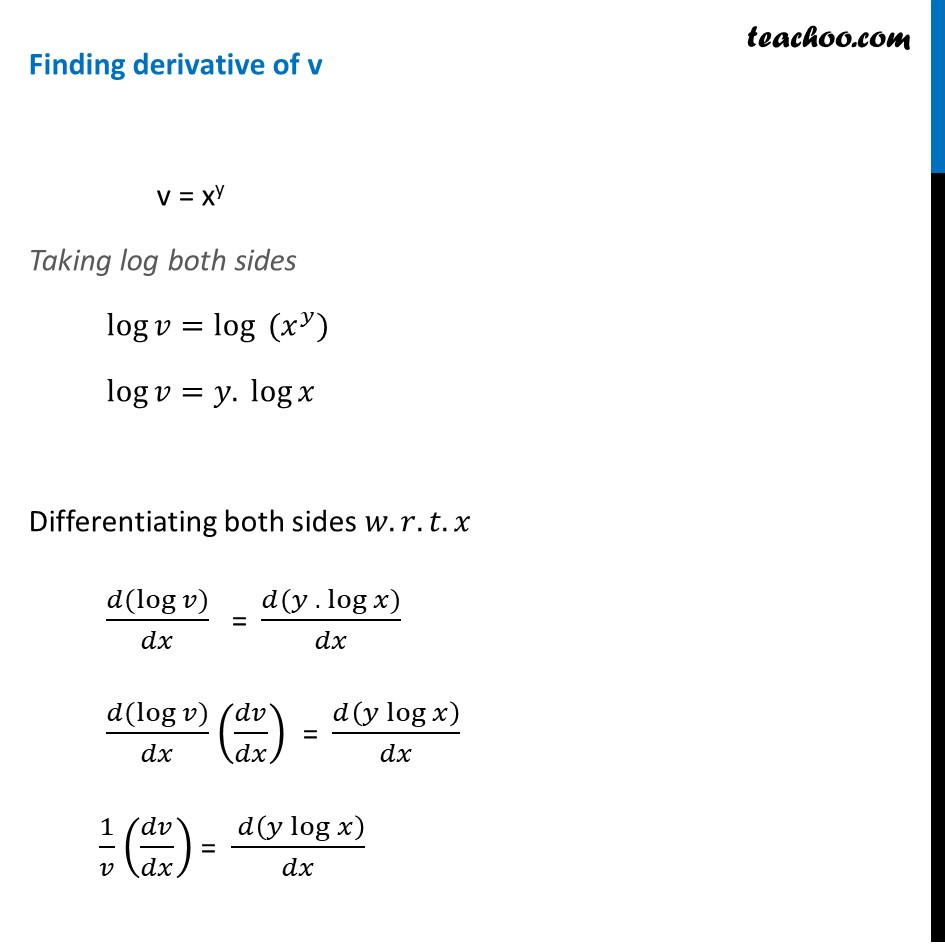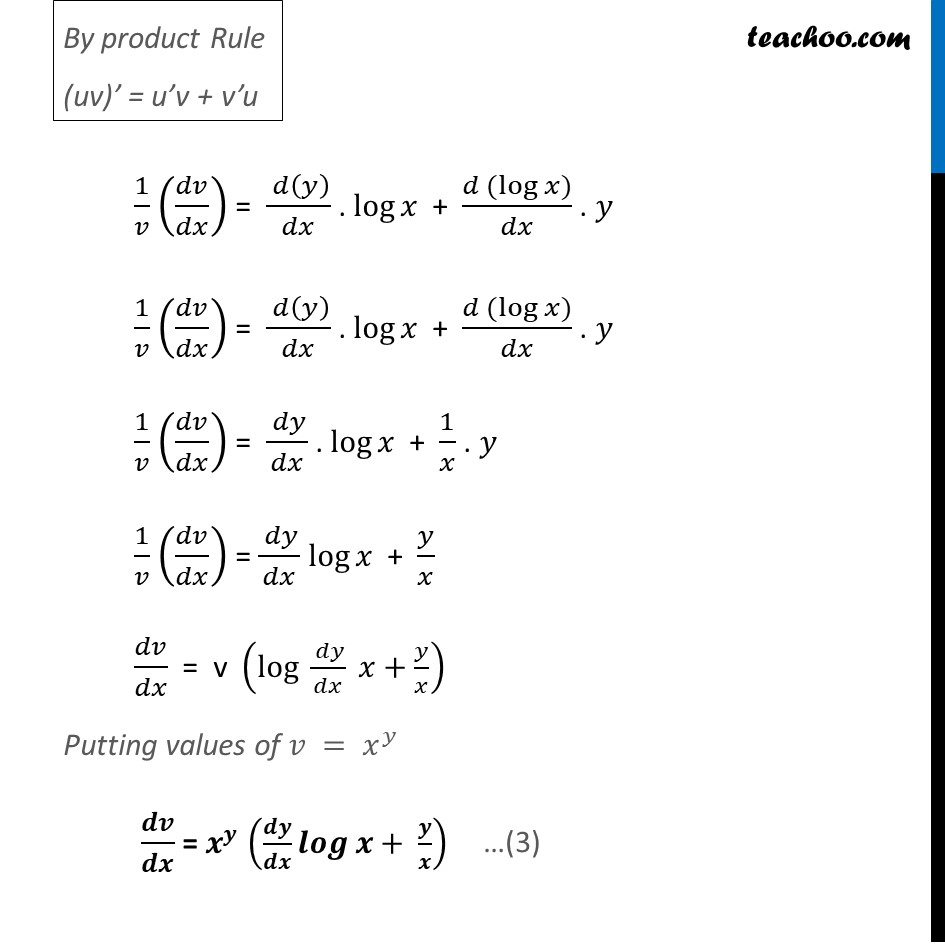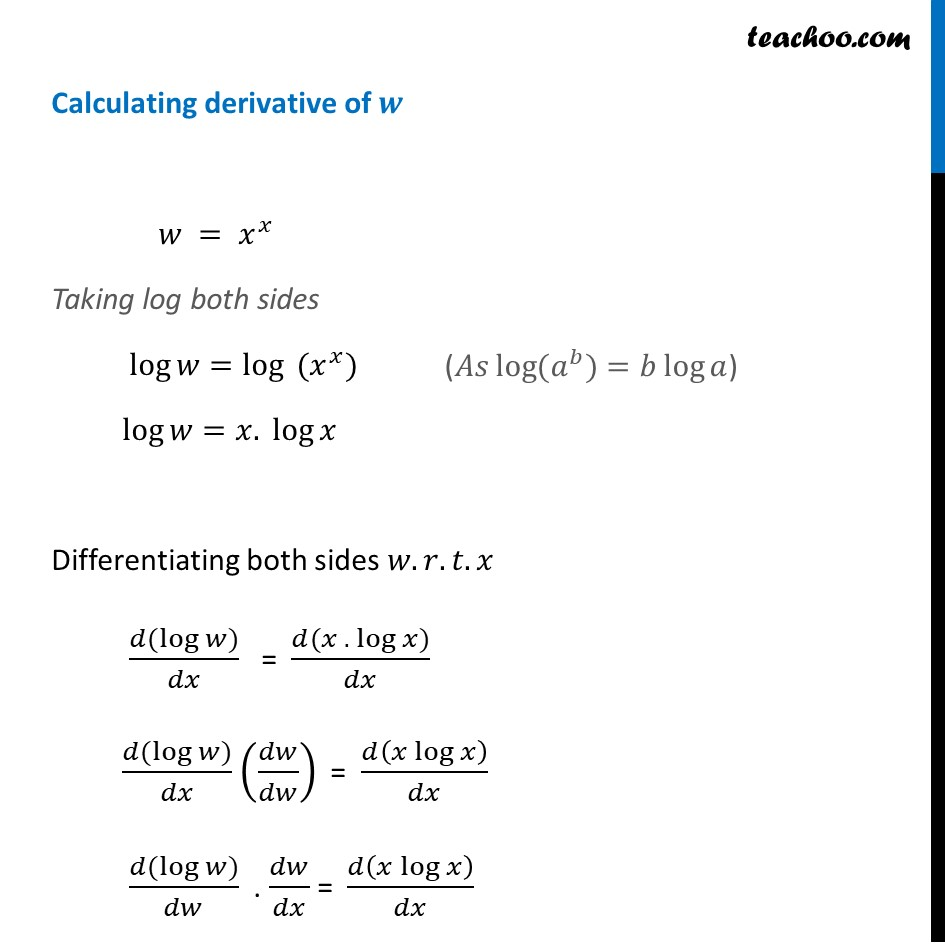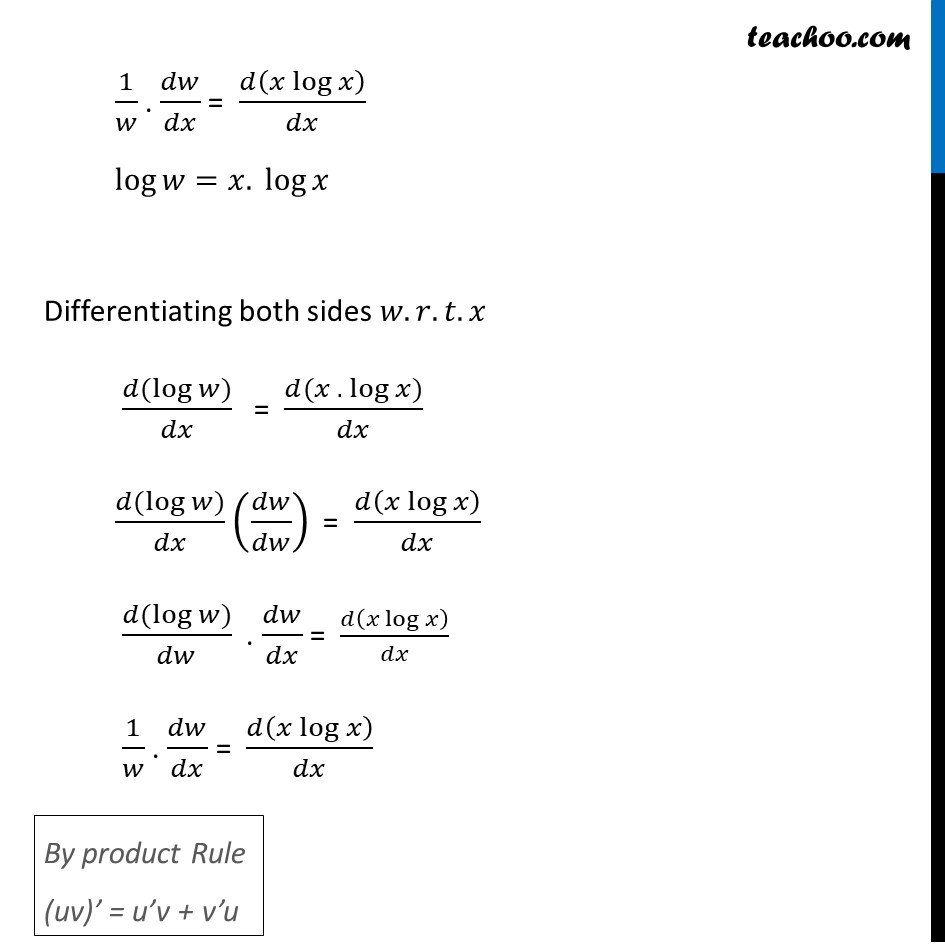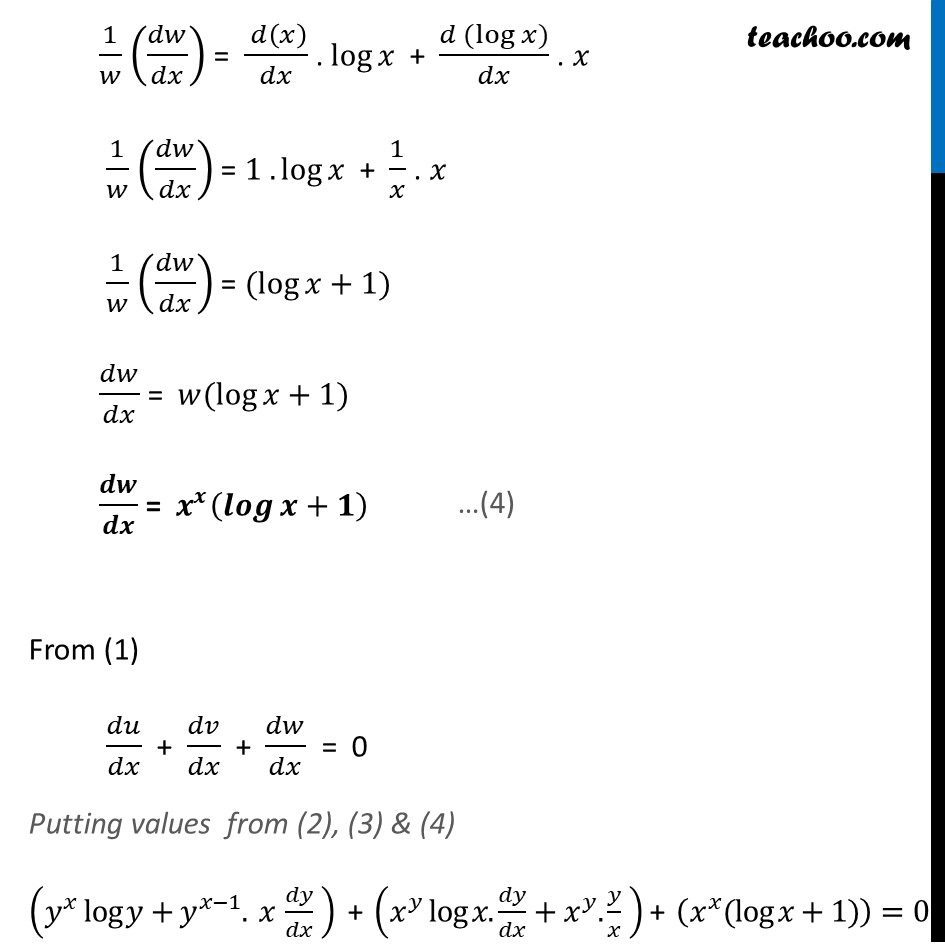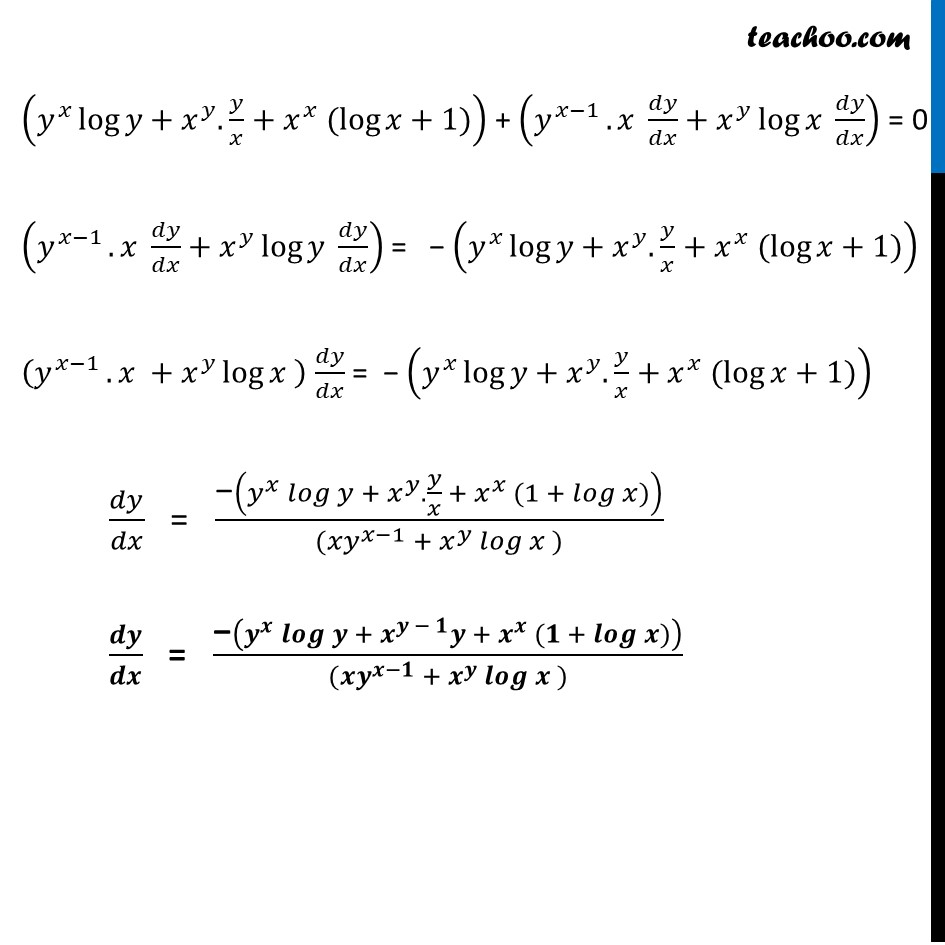Learn in your speed, with individual attention - Teachoo Maths 1-on-1 Class

### Transcript

Example 30 Find 𝑑𝑦/𝑑𝑥 , if 𝑦^𝑥+𝑥^𝑦+𝑥^𝑥=𝑎^𝑏. Let u = 𝑦𝑥, v = 𝑥𝑦 & w = 𝑥^𝑥 Now, 𝒖 + 𝒗 + 𝒘 = 𝒂^𝒃 Differentiating 𝑤.𝑟.𝑡.𝑥 (𝑑 (𝑢 + 𝑣 + 𝑤))/𝑑𝑥 = (𝑑(𝑎^𝑏))/𝑑𝑥 (𝑑(𝑢))/𝑑𝑥 + (𝑑(𝑣))/𝑑𝑥 + (𝑑(𝑤))/𝑑𝑥 = 0 We will calculate derivative of u, v & w separately . Finding Derivative of 𝒖 . 𝑢 = 𝑦^𝑥 Taking log both sides log⁡𝑢=log⁡〖 (𝑦^𝑥)" " 〗 log⁡𝑢=〖𝑥 . log〗⁡𝑦" " Differentiating both sides 𝑤.𝑟.𝑡.𝑥 (𝑑(log⁡𝑢))/𝑑𝑥 = (𝑑(𝑥 . log⁡𝑦))/𝑑𝑥 (𝑑(log⁡𝑢))/𝑑𝑥 (𝑑𝑢/𝑑𝑢) = 𝑑(𝑥.log⁡𝑦 )/𝑑𝑥 1/𝑢 . 𝑑𝑢/𝑑𝑥 = (𝑑 (𝑥 . log⁡𝑦 ))/𝑑𝑥 (𝐴𝑠 log⁡〖(𝑎^𝑏)〗=𝑏 log⁡𝑎) By product Rule (uv)’ = u’v + v’u 1/𝑢 . 𝑑𝑢/𝑑𝑥 = 𝑑𝑥/𝑑𝑥 . log⁡𝑦 + (𝑑(log⁡𝑦))/𝑑𝑥 . 𝑥 1/𝑢 . 𝑑𝑢/𝑑𝑥 = 1 . log⁡𝑦 + 𝑥. 𝑑(log⁡𝑦 )/𝑑𝑥 . 𝑑𝑦/𝑑𝑦 1/𝑢 . 𝑑𝑢/𝑑𝑥 = log⁡𝑦 + 𝑥. 𝑑(log⁡𝑦 )/𝑑𝑥 . 𝑑𝑦/𝑑𝑥 1/𝑢 . 𝑑𝑢/𝑑𝑥 = log⁡𝑦 + 𝑥. 1/𝑦 . 𝑑𝑦/𝑑𝑥 1/𝑢 . 𝑑𝑢/𝑑𝑥 = log⁡𝑦 + 𝑥/𝑦 . 𝑑𝑦/𝑑𝑥 𝑑𝑢/𝑑𝑥 = 𝑢 (log⁡𝑦 "+ " 𝑥/𝑦 " " 𝑑𝑦/𝑑𝑥) 𝒅𝒖/𝒅𝒙 = 𝒚^𝒙 (𝒍𝒐𝒈⁡𝒚 "+ " 𝒙/𝒚 " " 𝒅𝒚/𝒅𝒙) Finding derivative of v v = xy Taking log both sides log⁡𝑣=log⁡〖 (𝑥^𝑦)" " 〗 log⁡𝑣=〖𝑦. log〗⁡𝑥" " Differentiating both sides 𝑤.𝑟.𝑡.𝑥 (𝑑(log⁡𝑣))/𝑑𝑥 = (𝑑(𝑦 . log⁡𝑥))/𝑑𝑥 (𝑑(log⁡𝑣))/𝑑𝑥 (𝑑𝑣/𝑑𝑥) = 𝑑(〖𝑦 log〗⁡𝑥 )/𝑑𝑥 1/𝑣 (𝑑𝑣/𝑑𝑥) = ( 𝑑(〖𝑦 log〗⁡𝑥 ))/𝑑𝑥 By product Rule (uv)’ = u’v + v’u 1/𝑣 (𝑑𝑣/𝑑𝑥) = ( 𝑑(𝑦))/𝑑𝑥 . log⁡𝑥 + (𝑑 (log⁡𝑥))/𝑑𝑥 . 𝑦 1/𝑣 (𝑑𝑣/𝑑𝑥) = ( 𝑑(𝑦))/𝑑𝑥 . log⁡𝑥 + (𝑑 (log⁡𝑥))/𝑑𝑥 . 𝑦 1/𝑣 (𝑑𝑣/𝑑𝑥) = ( 𝑑𝑦)/𝑑𝑥 . log⁡𝑥 + 1/𝑥 . 𝑦 1/𝑣 (𝑑𝑣/𝑑𝑥) = ( 𝑑𝑦)/𝑑𝑥 log⁡𝑥 + 𝑦/𝑥 𝑑𝑣/𝑑𝑥 = v (log ( 𝑑𝑦)/𝑑𝑥 𝑥+𝑦/𝑥) Putting values of 𝑣 = 𝑥^𝑦 𝒅𝒗/𝒅𝒙 = 𝒙^𝒚 (𝒅𝒚/𝒅𝒙 𝒍𝒐𝒈⁡〖𝒙+ 𝒚/𝒙〗 ) Calculating derivative of 𝒘 𝑤 = 𝑥^𝑥 Taking log both sides log⁡𝑤=log⁡〖 (𝑥^𝑥)" " 〗 log⁡𝑤=〖𝑥. log〗⁡𝑥" " Differentiating both sides 𝑤.𝑟.𝑡.𝑥 (𝑑(log⁡𝑤))/𝑑𝑥 = (𝑑(𝑥 . log⁡𝑥))/𝑑𝑥 (𝑑(log⁡𝑤))/𝑑𝑥 (𝑑𝑤/𝑑𝑤) = 𝑑(𝑥 log⁡𝑥 )/𝑑𝑥 (𝑑(log⁡𝑤))/𝑑𝑤 . 𝑑𝑤/𝑑𝑥 = 𝑑(𝑥 log⁡𝑥 )/𝑑𝑥 (𝐴𝑠 log⁡〖(𝑎^𝑏)〗=𝑏 log⁡𝑎) 1/𝑤 . 𝑑𝑤/𝑑𝑥 = 𝑑(𝑥 log⁡𝑥 )/𝑑𝑥 log⁡𝑤=〖𝑥. log〗⁡𝑥" " Differentiating both sides 𝑤.𝑟.𝑡.𝑥 (𝑑(log⁡𝑤))/𝑑𝑥 = (𝑑(𝑥 . log⁡𝑥))/𝑑𝑥 (𝑑(log⁡𝑤))/𝑑𝑥 (𝑑𝑤/𝑑𝑤) = 𝑑(𝑥 log⁡𝑥 )/𝑑𝑥 (𝑑(log⁡𝑤))/𝑑𝑤 . 𝑑𝑤/𝑑𝑥 = 𝑑(𝑥 log⁡𝑥 )/𝑑𝑥 1/𝑤 . 𝑑𝑤/𝑑𝑥 = 𝑑(𝑥 log⁡𝑥 )/𝑑𝑥 By product Rule (uv)’ = u’v + v’u 1/𝑤 (𝑑𝑤/𝑑𝑥) = ( 𝑑(𝑥))/𝑑𝑥 . log⁡𝑥 + (𝑑 (log⁡𝑥))/𝑑𝑥 . 𝑥 1/𝑤 (𝑑𝑤/𝑑𝑥) = 1 . log⁡𝑥 + 1/𝑥 . 𝑥 1/𝑤 (𝑑𝑤/𝑑𝑥) = (log⁡〖𝑥+1〗) 𝑑𝑤/𝑑𝑥 = 𝑤(log⁡〖𝑥+1〗) 𝒅𝒘/𝒅𝒙 = 𝒙^𝒙 (𝒍𝒐𝒈⁡〖𝒙+𝟏〗 ) From (1) 𝑑𝑢/𝑑𝑥 + 𝑑𝑣/𝑑𝑥 + 𝑑𝑤/𝑑𝑥 = 0 Putting values from (2), (3) & (4) (𝑦^𝑥 log⁡〖𝑦+𝑦^(𝑥−1). 𝑥 𝑑𝑦/𝑑𝑥 〗 ) + (𝑥^𝑦 log⁡〖𝑥.𝑑𝑦/𝑑𝑥+𝑥^𝑦.𝑦/𝑥 〗 ) + (𝑥^𝑥 (log⁡〖𝑥+1〗))=0(𝑦^𝑥 log⁡〖𝑦+𝑥^𝑦. 𝑦/𝑥+𝑥^𝑥 (log⁡〖𝑥+1〗)〗 ) + (𝑦^(𝑥−1) .⁡〖𝑥 𝑑𝑦/𝑑𝑥+𝑥^𝑦 log⁡〖𝑥 𝑑𝑦/𝑑𝑥〗 〗 ) = 0 (𝑦^(𝑥−1) .⁡〖𝑥 𝑑𝑦/𝑑𝑥+𝑥^𝑦 log⁡〖𝑦 𝑑𝑦/𝑑𝑥〗 〗 ) = − (𝑦^𝑥 log⁡〖𝑦+𝑥^𝑦. 𝑦/𝑥+𝑥^𝑥 (log⁡〖𝑥+1〗)〗 ) (𝑦^(𝑥−1) .⁡〖𝑥 +𝑥^𝑦 log⁡〖𝑥 〗 〗 ) 𝑑𝑦/𝑑𝑥 = − (𝑦^𝑥 log⁡〖𝑦+𝑥^𝑦. 𝑦/𝑥+𝑥^𝑥 (log⁡〖𝑥+1〗)〗 ) 𝑑𝑦/𝑑𝑥 = "−" (𝑦^𝑥 𝑙𝑜𝑔⁡〖𝑦 + 𝑥^𝑦. 𝑦/𝑥 + 𝑥^𝑥 (1 + 𝑙𝑜𝑔⁡𝑥)〗 )/((〖𝑥𝑦〗^(𝑥−1) +⁡〖𝑥^𝑦 𝑙𝑜𝑔⁡〖𝑥 〗 〗)) 𝒅𝒚/𝒅𝒙 = "−" (𝒚^𝒙 𝒍𝒐𝒈⁡〖𝒚 + 𝒙^(𝒚 − 𝟏) 𝒚 + 𝒙^𝒙 (𝟏 + 𝒍𝒐𝒈⁡𝒙)〗 )/((〖𝒙𝒚〗^(𝒙−𝟏) +⁡〖𝒙^𝒚 𝒍𝒐𝒈⁡〖𝒙 〗 〗))